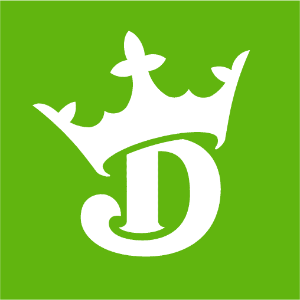DraftKings NL Championship Odds
+180
1.8 to 1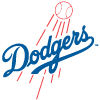Dodgers
29.8% implied probability

+600
6 to 1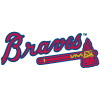Braves
11.9% implied probability

No changes have been recorded yet.
+900
9 to 1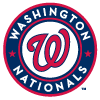Nationals
8.4% implied probability

+1000
10 to 1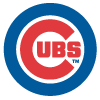Cubs
7.6% implied probability

+1000
10 to 1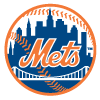Mets
7.6% implied probability

+1200
12 to 1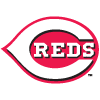Reds
6.4% implied probability

+1200
12 to 1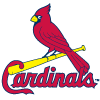Cardinals
6.4% implied probability

+1300
13 to 1Phillies
6.0% implied probability

+2000
20 to 1Brewers
4.0% implied probability

+2000
20 to 1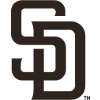Padres
4.0% implied probability

+2400
24 to 1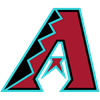Diamondbacks
3.3% implied probability

+3500
35 to 1Rockies
2.3% implied probability

+10000
100 to 1Pirates
0.8% implied probability

+10000
100 to 1Giants
0.8% implied probability

+15000
150 to 1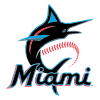Marlins
0.6% implied probability